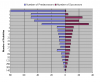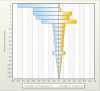## Recommended Posts

I have a chart that was created in Excel and I have been told to replicate it for the web using Fusion Charts.

I am running the latest FC version.

Image example attached.

Y Axis: Number of Activities

X Axis: Number of Predecessors OR Successors

The problem is that I need 0 to be the starting point and then the predecessors goes left (with increasing number) AND the successors goes right (with increasing number). Is this chart possible in FC? I have taken a look at the stacked bar chart but cannot figure out how to get two positive X Axis.

Thanks...##### Share on other sites

I have almost got what I want. Please view attached chart.

I now have only 1 thing I need to solve.

I was able to get the X Axis to go in two directions by using negative numbers.

Is there any way to "mask" the X Axis using maybe an absolute function so the numbers show up as positive?

One more thing, how do I get the bars to be solid colors??? I do not like gradients or alpha.

Thanks...##### Share on other sites

Hello,

Could you please attach the XML, we'd be glad to take a look and see how far we can tweak it.

##### Share on other sites

Sure, XML attached.

Thanks

StBar2D2.xml

##### Share on other sites

Hello? Any update on this?

Thanks...

##### Share on other sites

OK, well thanks for answering ONE of the two questions I posted several days ago...

The solution did not work by the way, I have attached the XML file for you to look at.

I am still seeing that ridiculous gradient. How do I remove it??

Also, please answer the other question I posted. Please read through the initial post and look at the attachments. I posted this several days ago.

StBar2D2.xml

##### Share on other sites

Hello, Could you please try with the following XML? Sorry for the delay, but I'm hoping this'll help!<chart showYAxisValues='0' divLineThickness='1' xaxisname="Number of Activities" animation="1" numDivLines="15" showValues="0" numVDivLines="15" palette="2" plotGradientColor=" ">

-

<categories>

<category label="1" showName="1"/>

<category label="1" showName="1"/>

<category label="1" showName="1"/>

<category label="1" showName="1"/>

<category label="1" showName="1"/>

<category label="3" showName="1"/>

<category label="12" showName="1"/>

<category label="5" showName="1"/>

<category label="22" showName="1"/>

<category label="22" showName="1"/>

<category label="30" showName="1"/>

<category label="27" showName="1"/>

<category label="5" showName="1"/>

<category label="69" showName="1"/>

<category label="93" showName="1"/>

<category label="123" showName="1"/>

<category label="256" showName="1"/>

<category label="303" showName="1"/>

<category label="145" showName="1"/>

</categories>

-

<dataset seriesName="Number of Predecessors" displayvalues='0'>

<set value="-80" hoverText="80"/>

<set value="-50"/>

<set value="-50"/>

<set value="-45"/>

<set value="-34"/>

<set value="-12"/>

<set value="-11"/>

<set value="-10"/>

<set value="-9"/>

<set value="-9"/>

<set value="-8"/>

<set value="-7"/>

<set value="-12"/>

<set value="-6"/>

<set value="-5"/>

<set value="-4"/>

<set value="-3"/>

<set value="-2"/>

<set value="-1"/>

</dataset>

-

<dataset seriesName="Number of Successors">

<set value="1"/>

<set value="4"/>

<set value="25"/>

<set value="20"/>

<set value="34"/>

<set value="12"/>

<set value="11"/>

<set value="10"/>

<set value="9"/>

<set value="8"/>

<set value="8"/>

<set value="7"/>

<set value="12"/>

<set value="6"/>

<set value="5"/>

<set value="4"/>

<set value="3"/>

<set value="2"/>

<set value="1"/>

</dataset>

<trendlines>

<line startValue='-10' displayValue='10'/>

<line startValue="-20" displayValue='20'/>

<line startValue="-30" displayValue='30'/>

<line startValue="-40" displayValue='40'/>

<line startValue="-50" displayValue='50'/>

<line startValue="-60" displayValue='60'/>

<line startValue="-70" displayValue='70'/>

<line startValue="-80" displayValue='80'/>

<line startValue="-90" displayValue='90'/>

<line startValue='0' displayValue='0'/>

<line startValue='10' displayValue='10'/>

<line startValue="20" displayValue='20'/>

<line startValue="30" displayValue='30'/>

<line startValue="40" displayValue='40'/>

<line startValue="50" displayValue='50'/>

<line startValue="60" displayValue='60'/>

<line startValue="70" displayValue='70'/>

<line startValue="80" displayValue='80'/>

<line startValue="90" displayValue='90'/>

</trendlines>

</chart>

##### Share on other sites

You guys are awesome! Works beautifully!

##### Share on other sites

Thanks! And you're most welcome.